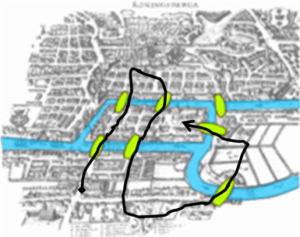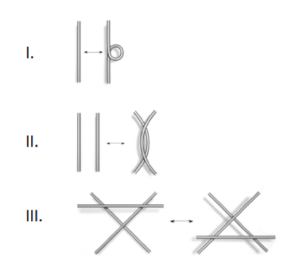Girls Talk Math is a non-traditional math camp in that students not only learn challenging Mathematics usually not encountered until college, but also research the life of female mathematicians who have worked on related topics. Campers share what they learned during the two-week day camp by writing a blog post about their Math topic and writing and recording a podcast about the mathematician they researched. Media created by the campers can be found on our website at www.girlstalkmath.web.unc.edu.

Girls Talk Math was founded in 2016 by Francesca Bernardi and Katrina Morgan, then Ph.D. candidates in Mathematics at UNC Chapel Hill. It was born of a desire to create a space for high-school students identifying as female or from an underrepresented gender who are interested in Mathematics. This summer, a sister camp at the University of Maryland at College Park had its first run thanks to Sarah Burnett and Cara Peters, Ph.D. candidates in Mathematics at UMD (www.gtm.math.umd.edu).

During two weeks of July 2018, 39 high schoolers came to the UNC Mathematics Department to participate in the 3rd year of Girls Talk Math. They were divided into groups of 4-5 campers, and each group completed a problem set focused on a different Math topic:

-Number Systems
-Network Science
-RSA Encryption Cryptography
-Elliptic Curve Cryptography
-Mathematical Epidemiology
-Quantum Mechanics
-Knot Theory
-Classification of Surfaces

Each group then wrote a blog post to share what they learned about their topic. Below are excerpts from each post written by the campers, and you can read the full blog posts here.

Number Systems
Miranda Copenhaver, Nancy Hindman, Efiotu Jagun, and Gloria Su

The Number Systems problem set focuses on learning about number bases (in particular, base 2 and 16) to understand how data is stored in computers and how to translate information into a language readable by machines. This problem set included coding in Python.

“[…]  We count in the decimal – or base 10 – system. This means that we count using 10 basic numbers: 0, 1, 2, 3, 4, 5, 6, 7, 8, and 9. In base 10, each place value represents a power of 10.”

“[…]  The two most important bases in coding are binary (base two) and hexadecimal (base sixteen). Binary is quite simple to think about because it only has two numbers that you could possibly use: 1 and 0.”

“[…]  If we had the number 1101010, we would start by labeling each place value with what power of two it represents. Next, we would multiply each digit by its power of two and simplify:

1101010 = 1*26 + 1*25 + 0*24 + 1*23 + 0*22 + 1*21 + 0*20

= 26 + 25 + 23 + 21

= 64 + 32 + 8 + 2

= 106

We see that the binary number 1101010 is the decimal number 106.”

Network Science
Myla James, Shania Johnson, Maya Mukerjee, and Savitha Saminathan

The Network Science problem set focuses on graph theory and how it is utilized for large data sets. Students learned about data storage in networks and how to analyze and study different data sets. This problem set included coding in Python.

“[…]  We were given a map of the city of Königsberg, Prussia that helped us learn about paths and circuits. Euler Paths and Circuits were named after Leonhard Euler, who asked the question: “Is there some route in this city wherein one would cross each of the seven bridges once and only once?”Königsberg, Prussia

“An Euler path must include two or less odd degree vertices. […]  In simplest form, an Euler path is a set of edges that is connected, and an Euler circuit is a set of edges that is connected and begins and ends at the same node. An analogy would be an electrical circuit. Electricity can flow in a closed circuit, but not an open path.”

RSA Encryption Cryptography
Camille Clark, Layke Jones, Isabella Lane, Aza McFadden, and Lizbeth Otero

The RSA Cryptography problem set introduces the field of Number Theory through modular arithmetic, prime numbers, and prime factorization. RSA cryptography is one of the most widespread methods to transmit codified information and has several applications in everyday technology.

“[…]  A common divisor is an integer that all the numbers in a given set can be divided into without a remainder. To calculate the of 2 numbers, you need to write out the prime factorization. (Camp directors’ note: the greatest common divisor (gcd) of two or more integers, not all zero, is the largest positive integer that divides each of the integers without a remainder.)

For example, let’s consider 8 and 12. The prime factorization of 8 is 23, while the prime factorization of 12 is 3*(22). Then, take the largest factor that overlaps in the two factorizations. Here, 22 is the largest factor in common between the prime factorizations of 8 and 12; then, 4 = gcd(8,12).

We say that two integers a and b are if gcd(a,b) = 1, where a and b don’t need to be prime themselves. For example, if a = 35 and b = 8, then gcd(a,b) = 1, but neither is a prime.”

Elliptic Curve Cryptography
Mukta Dharmapurikar, Anagha Jandhyala, Savanna Jones, and Ciara Renaud

In the Elliptic Curve Cryptography (ECC) problem set students learn how to apply this fascinating method of encoding, transmitting, and deciphering information. Elliptic Curve Cryptography is an interesting application of very theoretical concepts from Algebraic Geometry and Abstract Algebra.

“[…]  While the road to understanding Elliptic Curve cryptography was interesting and exciting, there were many twists and turns along the way. Our greatest challenge was that ECC is extremely hard to conceptualize as most of the math differed from our previous understandings and was often very theoretical or abstract.

However, we thoroughly enjoyed learning about topics in math, typically not discussed in school. For example, on the first day, we were learning about modular arithmetic. It was a difficult concept to grasp because it was fundamentally different than what we had learned before. Over time, just by working through the problem set, we became more and more comfortable with the topic, even going as far as being able to explain how it works to other people.

This goes to show, that even when faced with a very difficult problem set, if you keep persevering, eventually you will understand the math. Girls Talk Math has really taught us to never give up, and increased our confidence in learning higher level math.”

Mathematical Epidemiology
Camilla Fratta, Ananya Jain, Sydney Mason, Gabby Matejowsky, and Nevaeh Pinkney

The Mathematical Epidemiology problem set introduces the concept of modeling as a whole and in particular focuses on modeling disease spreading in populations. In this problem set campers have used an applet in Python.

“[…]  A mathematical model is an equation used to predict or model the most likely results to occur in a real-world situation. We used these types of equations to model the spread of a disease in a population, tracking the flow of populations from susceptible to infected to recovered. In real life scenarios, there are too many variables to fully account for, so we only were able to place a few in our equations. This made the models less accurate, but at the same time very useful to us in our problem set. They gave us a good idea of how things worked in an actual epidemic and helped us to understand what mathematical modeling really is.”

Quantum Mechanics
Izzy Cox, Divya Iyer, Wgoud Mansour, Ashleigh Sico, and Elizabeth Whetzel

The Quantum Mechanics problem set starts by explaining why classical mechanics does not describe properly the behavior of subatomic particles. It then introduces the main concepts of quantum mechanics, in particular focusing on the wave-particle duality, i.e. the fact that mass can be described as both a particle and a wave. As part of their problem set, campers ran a physical experiment to measure Planck’s Constant.

“[…] Quantum Mechanics is the physics of molecular and microscopic particles. However, it has applications in everyday life as well. If someone asked you if a human was a particle or a wave, what would you think? What about a ball? What about light? Not so easy now, is it? It turns out that all of those things, and in fact, everything around us, can be expressed in physics as both a particle and a wave.”

“[…] The realm of physics gets much stranger when it gets smaller! […]  [Quantum mechanics] is arguably one of the most complicated fields of physics, where all traditional rules are wrong. There is much still being added, and so much more to be discovered.”

Knot Theory
Jillian Byrnes, Monique Dacanay, Kaycee DeArmey, Alana Drumgold, Ariyana Smith, and Wisdom Talley

The Knot Theory problem set discusses the fascinating field of Abstract Geometry that deals with knots. Maybe surprisingly, there is a Mathematical theory behind tying and untying knots which can be described formally with algebraic symbols. This problem set is a Mathematical approach to knots and how to study and classify them.

“[…]  The Reidemeister moves are the three possible manipulations of knots that are used to find out if two diagrams represent equivalent knots. None of them physically change the knot, because they don’t make any cuts or make the knot intersect itself, so if the two diagrams are equivalent, they are related through a sequence of these three moves:”Three examples of Reidemeister moves: I, II, III

Classification of Surfaces
Ayanna Blake, Lisa Oommen, Myla Marve, Tamarr Moore, Caylah Vickers, and Lily Zeng

The Classification of Surfaces problem set deals with questions of shape, size, and the properties of space. Starting from a Mathematical definition of surfaces, students learn about aspects of a number of shapes, some of which they are already familiar with and some that do not exist in 3-dimensional space, with the aim of classifying them.

“[…]  Before we start off with explaining the basics, we give the definition of a surface, which is an example of a two-dimensional object. When talking about dimensions, basically it’s a way of classifying how many directions of travel an object has.

For example, a line on a piece of paper would be one dimensional because you can only go up or down on that line. A sheet of paper would be two dimensional because you can draw up or down and side to side. A room would be three dimensional because if you imagine throwing a ball in the air, it can move up or down, side to side, and forwards or backwards.”

* Girls Talk Math has been funded through the Mathematical Association of America Tensor Women and Mathematics grant which has supported the camp for the past three years. *

Peer edited by Rachel Cherney.

Follow us on social media and never miss an article: